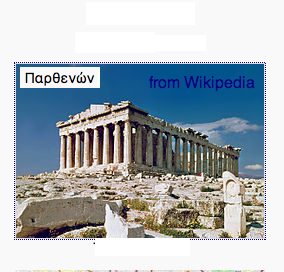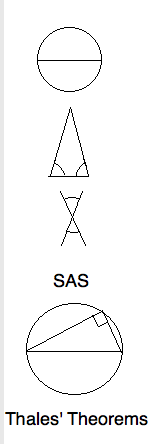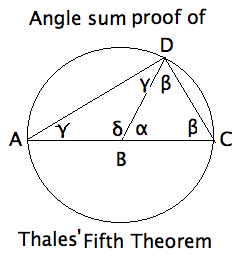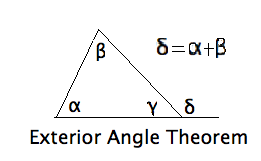Greek Geometry: Thales to Pappus
\textit{ $\C$ 2010, Prof. George K. Francis, Mathematics Department, University of Illinois}

revised 22aug11
\begin{document}
\section{Introduction}Thanks to the ubiquity of Google and other search engines, an enormous
source of information is at your finger tips at almost all of the time.
Much of it is overwhelming, and some of it is wrong. But
mostly, the result of undigested googling leads to misplaced emphases
and mental indigestion.
So here you will find comments on the text and lessons,
which you can always expand more fully
by googling the web. Think of this material as study guides.

\section{Origins of Greek Geometry}

Let start by setting up an easily remembered (approximate) timeline.

\begin{itemize}
\item[-900] Egypt, Mesopotamia, Persia, surveying and astronomy
\item[-600] Greece: Thales, Pythagoras
\item[-300] Euclid, Eudoxus
\item[-200] Archimedes, Apollonius
\item[+300] Pappus of Alexandria
\item[+800] Baghdad: algebra, preserves Euclidean geometry
\item[+1000] Europe: universities
\item[+1600] Newton, Leibniz, calculus
\item[+1800] Gauss, Lobachevsky, Bolyai, non-Euclidean geometry
\item[+1850] Riemann, Poincare, Klein, modern geometry
\item[+1900] Hilbert, end of the Parallel Postulate Problem.
\end{itemize}

\section{Thales' Theorems}

It is said that Thales knew proofs of five important theorems. His works are
lost, so we don't really know whether his proofs were correct. The five
theorems can be easily remembered by mnemonic figures.\begin{itemize}
\item  That the diameter bisects the circle seems too obvious to prove.
Perhaps Thales meant something more profound by the word bisects".
\item  A triangle is \textit{isosceles} if two of its sides are equal.
That this implies equal base angle isn't as obvious as the previous
theorem. In fact, Euclid
felt obliged to prove this in a very complicated way.
\item  Like the first two theorem, that vertical angles are equal is
another observation that can be deduced from symmetry. Not so the next two.
\item The Side-Angle-Side criterion for two triangles to be congruent
is a profound observation. Euclid's proof is incomplete. Indeed, Hilbert
takes this as an axiom, not because it is obvious, but because it is a
powerful place to start.
\item  That all triangles inscribed in a semicircle are right triangles
is generally just called \textit{Thales' Theorem.}
\end{itemize}

\section{Pappus' Proof of Pons Asinorum}
Thales' second theorem generally known by its medieval name,
\textit{pons asinorum} (bridge of asses), because stupid, or
stubborn  geometry students  could not master its proof.
In the early days of computers, MIT scientists programmed one
to discover and prove simple geometrical theorems. Most of the
theorems it discovered were uninteresting, as were its
deduction from the axioms and previous theorems it had discovered.
But when the computer rediscovered" Pappus' argument using SAS
the artificial intelligence community was mightly pleased. The
proof in the classical two column format might look like this:\begin{eqnarray*}
AB & \cong & AC & \mbox{given isosceles} \\
\angle BAC & \cong&  \angle CAB & \mbox{same angle} \\
AC&  \cong&  AB & \mbox{given isosceles} \\
\therefore \triangle BAC & \cong&  \triangle CAB & \mbox{by SAS} \\
\therefore \angle ABC&  \cong&  \angle ACB & \mbox{by congruence} \\
\end{eqnarray*}

Question 1.
Use ASA congruence to prove the converse of Pons Asinorum. Namely, that if
a triangle has a pair of congruent angles then it is isosceles. Recall that
ASA says if two triangles have two angles and the side between them in
one congruent to a corresponding triple in the other, then they are congruent.

\section{Hvidsten's Proof of Thales' Theorem}
In Sec. 1.2.2, the author of the popular text
"Geometry with Geometry Explorer" invites the reader
with a Try proving this theorem".
Such invitations are common in math books.
Coming this early in the text
suggests that somehow  not being able to supply the proof, a
student is unprepared for the course.
We'll interpret this as an
invitation to the teacher to elaborate, and derive some extra
mileage. However, such  internal exercises" should never be ignored.
They give the reader insight into the author's level of discourse.
Ignoring them leads to the common complaint by students that the
exposition is confusing". Whenever you are tempted to dismiss an
argument as simply confusing, you should reflect on, and formulate
just what you don't understand, and possibly why.

First, connect the vertex $D$ with the center $B$ of the
circle.
Compare the picture on the right with that in the text. Note that
the angles $\gamma, \beta$ are repeated by \textit{pons asinorum}
(both triangles are isosceles).The author suggests using the prior" theorem that the angles
of a triangle add up to $\pi$. Actually, this \textit{Angle Sum
Theorem} is equivalent to the \textit{Exterior Angle Theorem }
(or EXAT for short). It says that the exterior angle of
a triangle equals the sum of the opposite interior angles.
This theorem has many applications. In our figure,

\begin{eqnarray*}
\angle \alpha &=& 2 \angle \gamma \\
\angle \delta &=& 2 \angle \beta \\
\therefore \angle \alpha + \angle \delta &=& 2 (\angle \gamma + \angle \beta) \\
\therefore \pi &=& 2 (\angle \gamma + \angle \beta) \\
\therefore \frac{\pi}{2} &=& \angle ADC \\
\therefore \triangle ADC &  & \mbox { is a right triangle } \\
\end{eqnarray*}

Question 2.
Did you understand how this proof goes? Can you reproduce the proof using
entirely different letters? For instance, $\triangle PQR$, inscribed
in a semi-circle with diameter$PQ$ and center $C$. Instead of labelling
the angles, use the traditional notation, to show that $\angle PRQ = \pi/2$.

\section{Exterior Angle Theorems}
There are two exterior angle theorems in
\textit{Euclid's Elements}. The first just says that the
exterior angle of a triangle is greater than either of thetwo opposite interior angles.  The more precise form used
here is peculiarly Euclidean, and is false in non-Euclidean geometries.

\section{Archimedes' Angle Trisection}
Perhaps you have heard of the impossibility of trisecting an arbitrary angle
by ruler and compass. The ancients suspected it, but the theorem was not proved
until the 19th century, using modern algebraic methods. Cranks still circulate
solutions", but their efforts contribute nothing to mathematics.The Greeks did know that if additional tools were allowed, for example certain
curves, then trisections became possible. Recall that their rulers were considered
unmarked \textit{straight-edges}. Archimedes, the greatest mathematician and
physicist of the ancient world, showed that if you were allowed to make two
marks on a ruler, it is possible to trisect every angle.

In the figure, the given angle is on the right with the three angle marks.
I have omitted letter labels to
wean you away from these geometrical training wheels. Letter labels make it too easy just to check each
item stated. The real world comes without labels, and you should learn to
read" a figure without them. So, draw a circle about the given angle, and
extend one side of the angle to an entire diameter, and then extend this
diameters as far to the left as needed. Euclids second postulate says that
you can do this. Modern geometry considers all lines to be infinite anyway.
Now mark a segment on a straight-edge equal to the radius of the circle.
Place the marked ruler in such a way that it passes through the end of
the other side of the angle, crosses the circle and lands on the extended
diamater exactly one radius distance further on. In the figure three segments
the length of a radius are marked more heavily. They form the sides of
two isosceles triangles. By \textit{Pons Asinorum} and EXAT applied
two times, the smallest angle is one-third the size of the original angle.
The angle marks help see the relationships.

Question 3.
Did you follow this proof? You should write the four proofs in this lesson